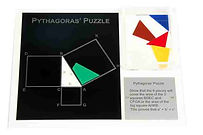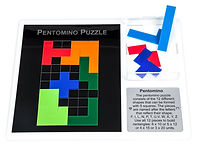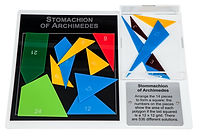top of page## MATHS PUZZLES5 Pieces, 6 Shapes

Use the five pieces to build either a triangle, or a square or a cross.  Can you also build a rectangle, a trapezium and a parralellogram?16 Squares, 5 Colours

Arrange the 16 squares (4 green, 3 red, 3 white, 3 blue and 3 yellow) in a 4 x 4 grid so that no colour appears more than once in any horizontal, vertical or diagonal line.Binary Puzzle

Arrange the 16 squares into a large 8x8 square so that each green square touches another green square and each yellow square touches another yellow square.Card Frame

Arrange the 10 cards to form a frame so that the number of diamonds on each side add up to 18.Hexagon Maze

Arrange the 19 hexagons so that there is a path from the start to the end going through each hexagon.  There are 3 hexagons with a straight path, 9 hexagons with a 60 turn and 7 hexagons with a 120 turn.Hinged Triangle

Arrange the 4 shapes to form a square then rearrange them to form a triangle. There are hinges at A, B and D.Magic Square

Arrange the numbers 1 to 9 in the 3 x 3 grid so that the sum of each row, column and diagonal is the same.

Magic Triangle

Arrange the numbers 1 to 9 on the triangle so that the sum of the numbers on each side of the triangle is the same.Pythagoras' Puzzle

Show that the 6 pieces will cover the area of the 2 squares BDEC and CFGA or the area of the big square AHKB.  This proves that a2 + b2 = C2Shunting Puzzle

Swap places:  move four yellow discs to the position of the 4 hexagons and the hexagons to where the yellow disks are – try to do this with as few moves as possible.  You may only move one piece at a time.Tangram Puzzle

Use the seven pieces, called “tans”, to build the shapes shown above.  All seven pieces have to be used and they may not overlap.Tower of Hanoi

Stack the discs neatly in order of size on one of the 3 pegs, smallest at top.  Move the entire stack to another peg, obeying the following rules:  Only one disc may be moved at a time.  No disc may be placed on top of a smaller disc.T-Puzzle

Arrange the four pieces to form the letter “T”.Triominos

Arrange the 21 Triominos (3 squares) and one 3 monomino (1 square) to form an 8x8 red and yellow chessboard.Arrow Loop Puzzle

Arrange the arrows in the 4x4 grid so that the path of the arrows forms a closed loop.  The dots indicate in which cell the next arrow must go.Pentomino

The pentomino puzzle consists of 12 different shapes that can be formed with 5 squares.  Use all 12 pieces to build different size rectangles.Stomachion of Archimedes

Arrange the 14 pieces to form a square.  There are 536 different solutionsTessellations

A Tessellation is a repeating pattern of polygons that cover an area without gaps or overlaps.  Use the diamonds or triangles to tile the area with one of the patterns shown or create your own design.

Tri-Rhombi Puzzle

Two equilateral triangles joined together, form a rhombus with all 4 sides the same length.  The 9 shapes are all different and make up of 3 rhombi.  Assemble the 9 shapes to form a hexagon.Magic Egg

Use the 9 pieces to build an egg.  Once you've done that, reassemble them to show what sort of bird could have hatched from it.  All 9 pieces have to be used and they may not overlap.Pythagoras' Puzzle

Show that the 6 pieces will cover the area of the 2 squares BDEC and CFGA or the area of the big square AHKB.  This proves that a2 + b2 = C2Seven Segment Puzzle

Arrange the numbers 0 to 9 in a 4 x 5 segment grid.

bottom of page